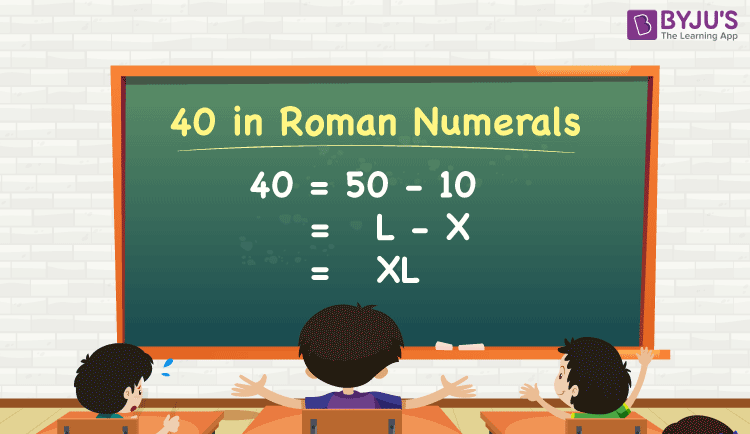# 40 in Roman Numerals

40 in Roman numerals is XL. One may get confused that 40 in Roman numerals is XXXX, but this is incorrect. Now the question is, why? To understand why we write 40 as XL in roman numerals, one should know the basic rules to convert the natural numbers to roman numerals, and vice versa. So, let’s have a look at the chart given below to know the equivalent roman numerals for some important numbers.

 Natural numbers 1 5 10 50 100 500 1000 Corresponding Roman numerals I V X L C D M

Learn: Roman numerals

## Conversion of 40 to Roman Numerals

The value of 40 in roman numerals is XL. The below figure shows the conversion of 40 to roman numerals.

##Also, try: Roman numerals Converter

## How to Write Numbers in Roman Numerals?

Consider the following sequence of roman numerals:

I, II, III, IV, V, VI, VII, VIII, IX, X, XI, XII, XIII, XIV, XV, XVI, XVII, XVIII, XIX, XX

From this, we can write the following basic rules for the conversion of roman numerals to numerical values.

• When a bigger letter precedes a smaller letter, we should subtract the corresponding numerical values. For example, the value of XL can be derived as L – X = 50 – 10 = 40, where L is the bigger number.
• Also, the notation XL can be read as “ten less than fifty, i.e. 40”.
• When a bigger letter succeeds a smaller letter, we should add the corresponding numerical values. Consider LX, where X is the bigger number. So, LX = L + X = 50 + 10 = 60. Also, the notation LX can be read as “ten more than fifty, i.e. 60”.

## Video Lesson on Roman Numerals### 1-40 in Roman Numerals

The below table shows the equivalent roman numerals for the numbers from 1 to 40.

 1 to 20 in Roman Numerals 21 to 40 in Roman Numerals Number Expansion Roman Numeral Number Roman Numeral 1 1 I 21 10 + 10 + 1 XXI 2 1 + 1 II 22 10 + 10 + 1 + 1 XXII 3 1 + 1 + 1 III 23 10 + 10 + 1 + 1 + 1 XXIII 4 5 – 1 IV 24 10 + 10 – 1 + 5 XXIV 5 5 V 25 10 + 10 + 5 XXV 6 5 + 1 VI 26 10 + 10 + 5 + 1 XXVI 7 5 + 1 + 1 VII 27 10 + 10 + 5 + 1 + 1 XXVII 8 5 + 1 + 1 + 1 VIII 28 10 + 10 + 5 + 1 + 1 + 1 XXVIII 9 10 – 1 IX 29 10 + 10 – 1 + 10 XXIX 10 10 X 30 10 + 10 + 10 XXX 11 10 + 1 XI 31 10 + 10 + 10 + 1 XXXI 12 10 + 1 + 1 XII 32 10 + 10 + 10 + 1 + 1 XXXII 13 10 + 1 + 1 + 1 XIII 33 10 + 10 + 10 + 1 + 1 + 1 XXXIII 14 10 – 1 + 5 XIV 34 10 + 10 + 10 – 1 + 5 XXXIV 15 10 + 5 XV 35 10 + 10 + 10 + 5 XXXV 16 10 + 5 + 1 XVI 36 10 + 10 + 10 + 5 + 1 XXXVI 17 10 + 5 + 1 + 1 XVII 37 10 + 10 + 10 + 5 + 1 + 1 XXXVII 18 10 + 5 + 1 + 1 + 1 XVIII 38 10 + 10 + 10 + 5 + 1 + 1 + 1 XXXVIII 19 10 – 1 + 10 XIX 39 10 + 10 + 10 – 1 + 10 XXXIV 20 10 + 10 XX 40 50 – 10 XL

Get the conversion of some specific numbers to Roman Numerals:

30 in Roman numerals = 10 + 10 + 10 = X + X + X = XXX

41 in Roman numerals = 50 – 10 + 1 = L – X + I = XLI

44 in Roman numerals = 50 – 10 – 1 + 5 = L – X – I + V = XLIV

45 in Roman numerals = 50 – 10 + 5 = L – X + V = XLV

49 in Roman numerals = 50 – 10 – 1 + 10 = L – X – I + 10 = XLIX

50 in Roman numerals = L

60 in Roman numerals = 50 +10 = L + X = LX

90 in Roman numerals = 100 – 10 = C – X = XC

500 in Roman numerals = D

1000 in Roman numerals = M